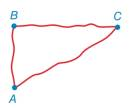Chapter 1.3, Problem 8E### Elementary Geometry for College St...

6th Edition
Daniel C. Alexander + 1 other
ISBN: 9781285195698

#### Solutions

Chapter
Section### Elementary Geometry for College St...

6th Edition
Daniel C. Alexander + 1 other
ISBN: 9781285195698
Textbook Problem
3 views

# A cross-country runner jogs at a rate of 15 feet per second. If she runs 300 feet from A to B, 450 feet from B to C, and then 600 feet from C back to A. how long will it take her to return to point A? See the figure for Exercise 7.Exercises 7, 8

To determine

To find:

Explanation

Given:

The given figure is,

A cross-country runner jogs at a rate of 15 feet per second. If she runs 300 feet from A to B, 450 feet from B to C, and then 600 feet from C back to A.

Calculation:

Consider the given figure,

Figure (1)

Distance covered by her from A to B is 300 feet.

Distance covered by her from B to C is 450 feet.

Distance covered by her from C to A is 600 feet.

Total distance covered by her is,

### Still sussing out bartleby?

Check out a sample textbook solution.

See a sample solution

#### The Solution to Your Study Problems

Bartleby provides explanations to thousands of textbook problems written by our experts, many with advanced degrees!

Get Started

#### Let f be the function defined by f(x) = 2x + 5. Find f(a + h), f(a), f(a2), f(a 2h), and f(2a h).

Applied Calculus for the Managerial, Life, and Social Sciences: A Brief Approach

#### Find the value of the sum. 34. i=1ni(i+1)(i+2)

Single Variable Calculus: Early Transcendentals, Volume I

#### Evaluate the integral. 31. 1+x1xdx

Single Variable Calculus: Early Transcendentals

#### Find for y defined implicity by .

Study Guide for Stewart's Multivariable Calculus, 8th